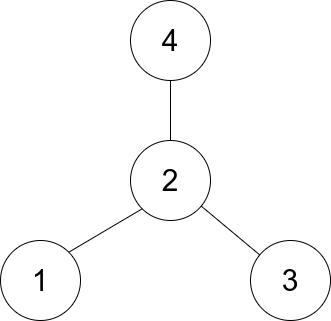1791. Find Center of Star Graph

#### QUESTION:

There is an undirected star graph consisting of n nodes labeled from 1 to n. A star graph is a graph where there is one center node and exactly n - 1 edges that connect the center node with every other node.

You are given a 2D integer array edges where each edges[i] = [ui, vi] indicates that there is an edge between the nodes ui and vi. Return the center of the given star graph.

Example 1:``````
Input: edges = [[1,2],[2,3],[4,2]]
Output: 2
Explanation: As shown in the figure above, node 2 is connected to every other node, so 2 is the center.
``````

Example 2:

``````Input: edges = [[1,2],[5,1],[1,3],[1,4]]
Output: 1
``````

Constraints:

``````3 <= n <= 105
edges.length == n - 1
edges[i].length == 2
1 <= ui, vi <= n
ui != vi
The given edges represent a valid star graph.
``````

#### SOLUTION:

``````class Solution {
fun findCenter(edges: Array<IntArray>): Int {
var indexs = IntArray(edges.size+2)
edges.forEach { array ->
indexs[array]++
indexs[array]++
}
var result = IntArray(2);
indexs.forEachIndexed { index, i ->
if (i > result) {
result = index
result = i
}
}
return result
}
}
``````
>